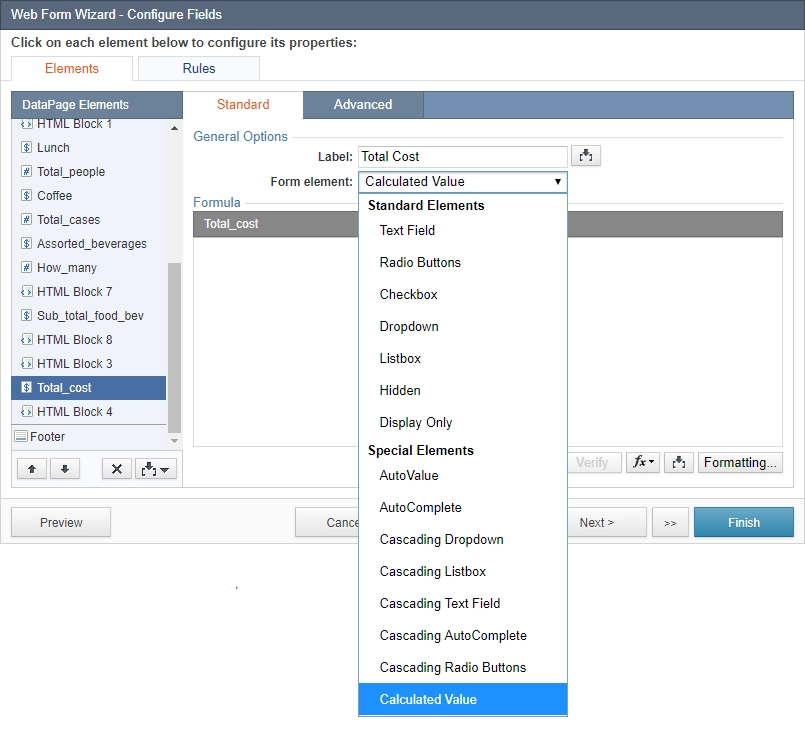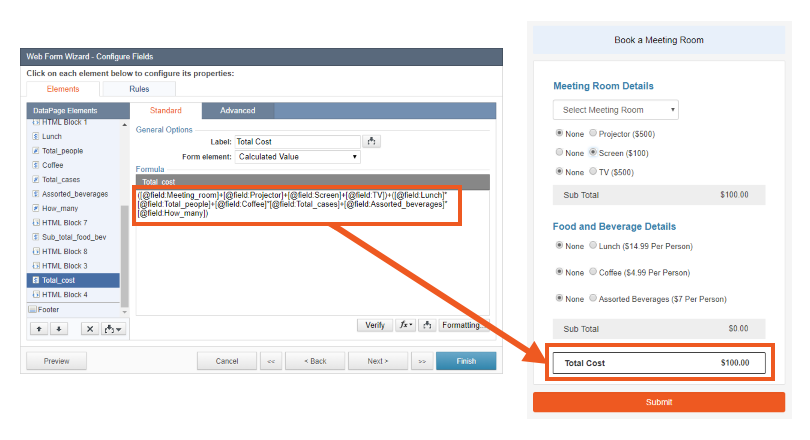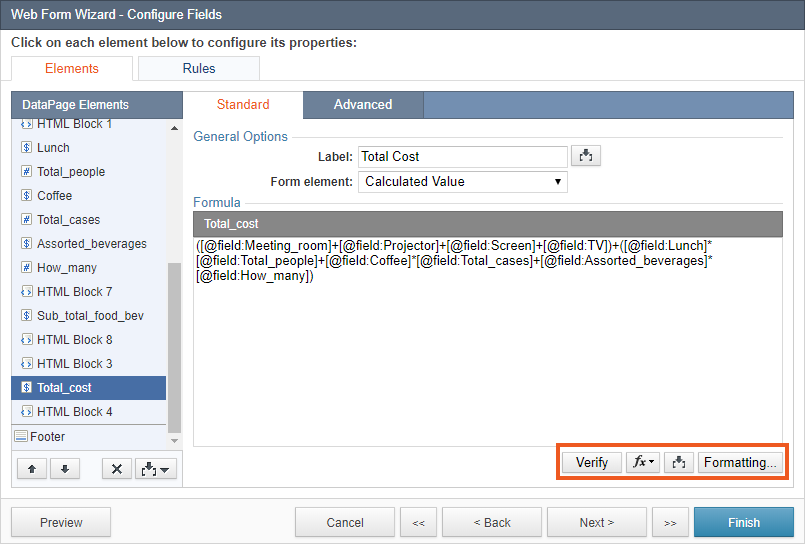The Calculated Value form element generates dynamic calculations that automatically update as users interact with your form. Similar to Formula Fields and Calculated Fields, you can construct the calculation using functions, logic, constants, values from other fields and SQL expressions.

Note: The Calculated Values option is available if the Formulas and Calculations feature is included in your plan.

To create a calculated value field, select a field under the DataPage Elements panel in the DataPage wizard and select Calculated Value in the Form element dropdown.Enter your formula or expression for the selected field in the Formula box. In the example below, a total cost is calculated based on the user's selections when booking a meeting room.

```([@field:Meeting_room]+[@field:Projector]+[@field:Screen]+[@field:TV])+([@field:Lunch]*[@field:Total_people]+[@field:Coffee]*[@field:Total_cases]+[@field:Assorted_beverages]*[@field:How_many])
```

Here is the DataPage displaying the total cost of each meeting room request:At the bottom right of the Formula panel, there are several options to help you create a formula.Formula options:

• To verify your formula, click the Verify button to confirm that the formula is valid.

Note: “Valid formula” is displayed if the formula or expression is valid. Otherwise, an error message is displayed.

• To view and select a function, click theFunction button. See Caspio’s Function Reference for a list of common functions.
• You can use theInsert button to add fields and parameters to use with formulas. These parameters will be dynamically replaced by actual data from the record at run time.
• To include Unicode characters such as extended Latin characters, symbols, emoji or non-Latin characters in the formula, add the N prefix to the string, for example: N’Ausländer’. Some Unicode characters are ignored in comparisons.
• The Formatting button allows you to choose the display format of the calculated value. You can assign the formatting from the localization of the DataPage, or specify custom formatting.

Calculated Value form elements support a variety of data types (i.e. number, string, date), so it must be configured properly to match the target field’s data type.